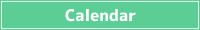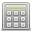<< < Oct. 2023 > >>
SunMonTueWedThuFriSat
1 2 3 4 5 6 7
8 9 10 11 12 13 14
15 16 17 18 19 20 21
22 23 24 25 26 27 28
29 30 31 1 2 3 4## Probability of sum of dice

### The sum of the probability of the dice when you shake 3 6 sided dice

The sum of the diceCombination(kinds)ProbabilityProbability(%)
3 1 0.0046296296296296 0.46296296296296
4 3 0.013888888888889 1.3888888888889
5 6 0.027777777777778 2.7777777777778
6 10 0.046296296296296 4.6296296296296
7 15 0.069444444444444 6.9444444444444
8 21 0.097222222222222 9.7222222222222
9 25 0.11574074074074 11.574074074074
10 27 0.125 12.5
11 27 0.125 12.5
12 25 0.11574074074074 11.574074074074
13 21 0.097222222222222 9.7222222222222
14 15 0.069444444444444 6.9444444444444
15 10 0.046296296296296 4.6296296296296
16 6 0.027777777777778 2.7777777777778
17 3 0.013888888888889 1.3888888888889
18 1 0.0046296296296296 0.46296296296296

## How to calculate the probability of sum of dice

To find the probability of the sum of the dice, find the number of all possible cases that yields the desired sum.

Consider the probability of rolling 3 six-sided dice and getting a sum of 5.

If you roll three 6-sided dice, the total number of possibilities is 6 x 6 x 6 = 216.

(1,1,3) (1,2,2) (1,3,1) (2,1,2) (2,2,1) (3,1,1) sums to 5 , so the probability is 6 ÷ 216 = 0.0277…

###Simple Calculator

Save results in a temporary